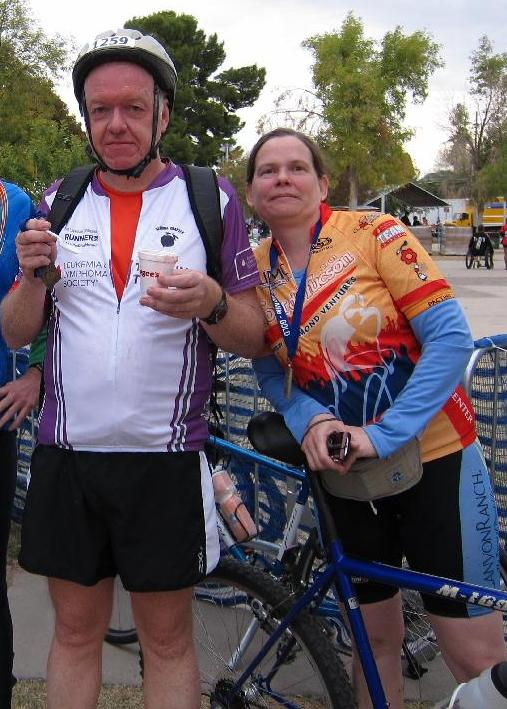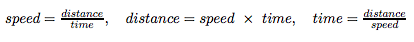# Mean Questions With Harmonious Answers

Aodh and Bea each biked half of a 180-kilometer fundraiser race, in that order, as a relay team. His average speed was 36 kilometers per hour, and hers was 20 kilometers per hour. What was their average speed overall?
This post was published on the now-closed HuffPost Contributor platform. Contributors control their own work and posted freely to our site. If you need to flag this entry as abusive, send us an email.Aodh and Bea each biked half of a 180-kilometer fundraiser race, in that order, as a relay team. His average speed was 36 kilometers per hour, and hers was 20 kilometers per hour. What was their average speed overall?

They shared the task of writing the thank-you notes to all their sponsors, a job that would take him three hours to do alone, but she could dash the notes off solo in two hours. Assuming that they started and finished at the same time, how long did it take them working "in tandem" together?

Incidentally, they paid \$75 on average to enter the race, he paying the maximum \$100 entry fee the day before the race, and she getting the early bird special rate of \$50 by registering months ahead of time.

The following table lists the above numerical information. The goal is to fill in the appropriate missing averages in the last row. (The final desired average would help with the question about the joint note writing time.)

It's tempting to conclude that their average speed was (36+20)/2 = 28 kilometers per hour, and also that, on average, it takes each of them 2.5 hours to write the thank-you notes, in which case it would only take 1.25 hours to do the job together. Those conclusions are incorrect, however. Our averaging is off in subtle ways, which we now explore carefully.

By "their average speed' we mean the constant speed at which a single person would have to ride to start as Aodh starts and finish alongside Bea. First recall the key relationships between average speed s, distance covered d and time taken t, namely s = d/t, or equivalently, by cross-multiplying, d = s x t, which can also be written as t = d/s. In summary:Aodh took 90/36 = 2.5 hours to ride the first 90 km, and Bea took 90/20 = 4.5 hours to finish the race. Overall, assuming a smooth "passing of the baton" at the halfway mark, it took this team 2.5 + 4.5 = 7 hours to complete the course. Had a single person ridden at 28 kilometers per hour for 7 hours, they'd have covered 28 x 7 = 196 kilometers, which is quite an overshoot, as the route is only 180 kilometers long. Where did we do wrong?

It's less obvious that the time estimate for cooperative thank-you note writing is also mistaken, but we'll get back to that later.

Let's take a closer look at the average speed question. Since the two of them rode for 7 hours overall, then a single person cycling at 180/7 (about 25.71) kilometers per hour for 7 hours covers 180 kilometers. The correct average of 35 and 20 here is thus roughly 25.71, not 28! Note that had we "averaged denominators, not numerators," we could have argued that on average each of them rode 90 kilometers in (2.5 + 4.5)/2 = 3.5 hours, and 90/3.5 also comes out to 25.72 kph. We'll return to this theme shortly.

Now we turn to the thank-you note writing. For the sake of definiteness, let's assume that they had 30 sponsors between them. (The exact number is not important; what follows will lead to the same conclusion if we change that to 15 or 60.)Because Aodh can write all 30 notes in 3 hours, on average he does 10 per hour; thus it takes him 6 minutes for each one. Bea, meanwhile, can do the job alone in 2 hours, so her rate is 15 per hour, hence 4 minutes apiece. So in a 12-minute period, he'd write two, and she'd write three, so, between them, they'd write five thank-you notes total. (Why consider 12? It's the least common multiple of 6 and 4. That came in handy the last time, too.)

Because we want 30 notes written, and 30 = 5 x 6, let's look at what happens in a 12 x 6 = 72-minute period. He'd write 2 x 6 = 12 and she'd write 3 x 6 = 18. Perfect! They finish at the same time, and all 12 + 18 = 30 notes are written as desired. So the correct answer to the second question is that it would take Aodh and Bea 1 hour and 12 minutes (not 1 hour and 15 minutes) to write the thank-you notes together.

What is going on here is that a type of average known as the harmonic mean underlies the correct approach to both questions. Surprisingly, it's not commonly encountered by students of mathematics, yet we're about to see that it arises naturally in real life.

The harmonic mean of two numbers A and B is the flip (reciprocal) of the average of the flips of A and B! More formally, it's:

A little algebraic manipulation simplifies this to the less scary-looking 2AB / (A + B). For A = 36 and B = 20 we get 2(36 x 20) / (36 + 20) = 25.71, the speed average found earlier.

The harmonic mean is appropriate when "the action is in the denominators," which is the case when comparing 90 kilometers covered in 2.5 hours with the same distance covered in 4.5 hours.

(Had Aodh instead cycled 90 kilometers in 3 hours and Bea covered 60 kilometers in the same amount of time, which yields average speeds of 30 kilometers per hour and 20 kilometers per hour, respectively, it would be fair to use the arithmetic mean and conclude that their average speeds were 25 kilometers per hour. That's a standard "numerator" averaging problem.)

Note that both the speed and note-writing information given above were in the form of rates, where the same fixed amount of something (distance, notes) was processed in various amounts of time. The first tricky question above turned out to tie in with a harmonic mean. What about the second one?

The harmonic mean of the times it takes Aodh and Bea to write the thank you notes, namely 3 and 2 hours, is 2(3 x 2) / (3 + 2) = 2.4 hours. That's how long, on average, it takes one of them to do the job. Doing it together halves that to 1.2 hours, or 72 minutes, agreeing with the answer found above, but with a lot less work.Similarly, if it takes 6 hours for pump A to fill a swimming pool, and 12 hours for pump B to do the same job, then working together the two pumps would fill the pool in 4 hours. (Check that the harmonic mean of 6 and 12 is 8.) This is another "working in parallel" problem, for which the harmonic mean is a natural fit. The note writing was such an exercise, despite the ambiguous use of the phrase "in tandem."

The harmonic mean also has many applications in physics, and when working with the liters-per-100-kilometers version of fuel efficiency for cars, and price-to-earnings ratio in economics.

Here's our final challenge: Chris decides to do the whole 180-kilometer ride solo. He sets himself the goal of averaging 30 kilometers per hour overall. He slacks off for the first half and only goes 15 kilometers per hour on average. How fast does he have to go in the second half to hit his target average?

This piece was inspired by a Mind Your Decisions puzzle by @preshtalwalkar. Thanks to Scott Anderson of Wellesley College for helpful discussions and for reminding us of the classic final challenge above. We trust that by now readers have guessed how the names are pronounced. It's as easy as Aodh, Bea, see? All images used with permission. (Thanks to Niall for the cyclists image.)

Close